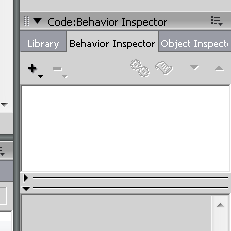Text and field behaviors for copy, paste, cut, and select all functions

Use Lingo to manipulate text data
This TechNote provides four example behaviors that perform standard Cut, Copy, Paste, and Select All functions. The behaviors are designed to be attached to editable field or text sprites.

For these behaviors to function, there must be a field cast member called "clipboard temp."

Note: These behaviors only work in projectors. They do not work in the authoring environment because Director traps control/command modified keys for its own uses. The same is true for Shockwave movies running in a browser; browser applications also trap control/command keys.

Example behaviors

To add the code, navigate to Behavior Inspector and add the snippet.

Run the code.Cut

The following behavior copies selected text when Command/Control+X has been detected:

property spriteNum, pWhichSprite, pWhichMember, pMemberType

on beginSprite me
pWhichSprite = sprite(me.spriteNum)
pWhichMember = pWhichSprite.member
pMemberType = pWhichMember.type
end

on keyDown me
if (the commandDown) then
case (TRUE) of
(the commandDown AND the keyPressed = "x") : clipboardCut me
end case
else
pass
end if
end

on clipboardCut me
if pMemberType = #text then
whichSelection = member(pWhichMember).selection
if whichSelection + 1 <> whichSelection then
put pWhichMember.selectedText.text into member("clipboard temp")
delete member(pWhichMember).char[whichSelection + 1..whichSelection]
copyToClipboard member "clipboard temp"
else
beep
end if
end if
if pMemberType = #field then
whichSelection = the selection
if the selStart<> the selEnd then
put the selection into member("clipboard temp")
a = the selStart
b = the selEnd
put char a + 1 to b of the text of member pWhichMember into member("clipboard temp")
delete char a + 1 to b of member pWhichMember
copyToClipboard member "clipboard temp"
else
beep
end if
end if
end

Copy

The following behavior copies selected text when Command/Control+C has been detected:

property spriteNum, pWhichSprite, pWhichMember, pMemberType
on beginSprite me
pWhichSprite = sprite(me.spriteNum)
pWhichMember = pWhichSprite.member
pMemberType = pWhichMember.type
end
on keyDown me
if (the commandDown) then
case (TRUE) of
(the commandDown AND the keyPressed = "c") : clipboardCopy me
end case
else
pass
end if
end
on clipboardCopy me
if pMemberType = #text then
if pWhichMember.selectedText.text <> "" then
put pWhichMember.selectedText.text into member("clipboard temp")
copyToClipboard member "clipboard temp"
else
beep
end if
end if
if pMemberType = #field then
if the selStart <> the selEnd then
a = the selStart
b = the selEnd
put char a + 1 to b of the text of member pWhichMember into member("clipboard temp")
copyToClipboard member "clipboard temp"
end if
end if
end

Paste

The following behavior pastes text when Command/Control+V has been detected:

property spriteNum, pWhichSprite, pWhichMember, pMemberType
on beginSprite me
pWhichSprite = sprite(me.spriteNum)
pWhichMember = pWhichSprite.member
pMemberType = pWhichMember.type
end
on keyDown me
if (the commandDown) then
case (TRUE) of
(the commandDown AND the keyPressed = "v") : clipboardpaste me
end case
else
pass
end if
end
on clipboardPaste me
clipBoardRef = member "clipboard temp"
pasteClipBoardInto member "clipboard temp"
clipBoardRef.name = "clipboard temp"
charCount = member("Clipboard temp").char.count
if pMemberType = #text then
whichSelection = member(pWhichMember).selection
num1 = whichSelection
num2 = whichSelection
if num1 <> num2 then
if num1 = 0 then
member(pWhichMember).char[1..num2] = member("Clipboard temp").text
member(pWhichMember).selection = [0, charCount]
else
member(pWhichMember).char[(num1 + 1)..num2] = member("Clipboard temp").text
member(pWhichMember).selection = [num1, (num1 + charCount)]
end if
else
if num1 = 0 then
x = member(pWhichMember).char
member(pWhichMember).char = member("Clipboard temp").text & x
member(pWhichMember).selection = [0, charCount]
else
x = member(pWhichMember).char[num1]
member(pWhichMember).char[num1] = x & member("Clipboard temp").text
member(pWhichMember).selection = [num1, (num1 + charCount)]
end if
end if
end if
if pMemberType = #field then
num1 = the selStart
num2 = the selEnd
if num1 <> num2 then
if num1 = 0 then
member(pWhichMember).char[1..num2] = member("Clipboard temp").text
the selStart = 0
the selEnd = charCount
else
member(pWhichMember).char[(num1 + 1)..num2] = member("Clipboard temp").text
the selStart = num1
the selEnd = (num1 + charCount)
end if
else
if num1 = 0 then
x = member(pWhichMember).char
member(pWhichMember).char = member("Clipboard temp").text & x
the selStart = 0
the selEnd = charCount
else
x = member(pWhichMember).char[num1]
member(pWhichMember).char[num1] = x & member("Clipboard temp").text
the selStart = num1
the selEnd = (num1 + charCount)
end if
end if
end if
end

Select All

The following behavior selects all text when Command/Control+A has been detected:

property spriteNum, pWhichSprite, pWhichMember, pMemberType
on beginSprite me
pWhichSprite = sprite(me.spriteNum)
pWhichMember = pWhichSprite.member
pMemberType = pWhichMember.type
end
on keyDown me
if (the commandDown) then
case (TRUE) of
(the commandDown AND the keyPressed = "a") : selectAll me
end case
else
pass
end if
end
on selectAll me
if pMemberType = #text then
num = member(pWhichMember).text.char.count
set the keyBoardFocusSprite = -1
end if
if pMemberType = #field then
set the keyBoardFocusSprite = -1
hilite member pWhichMember
end if
end